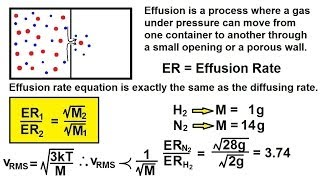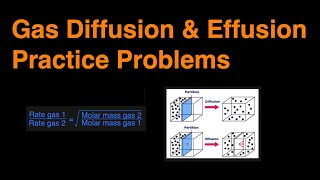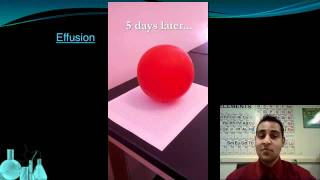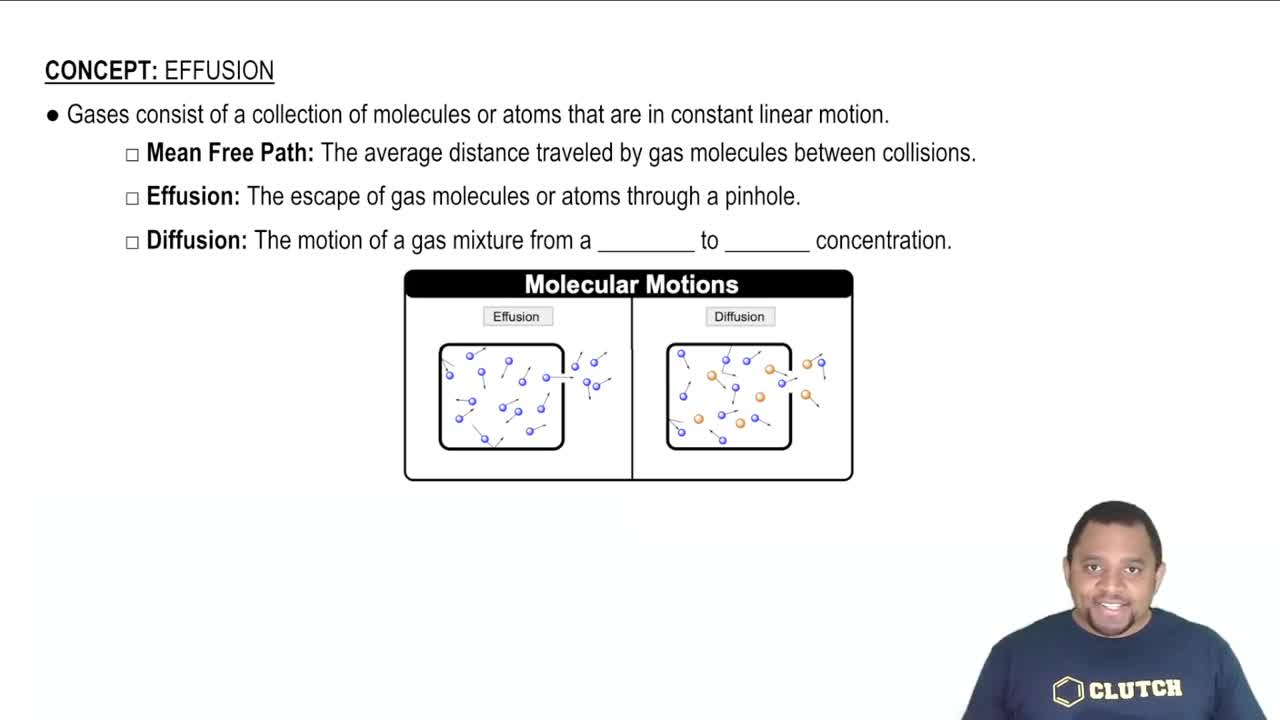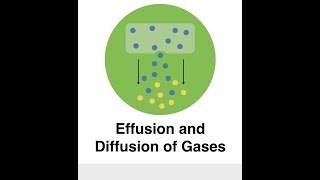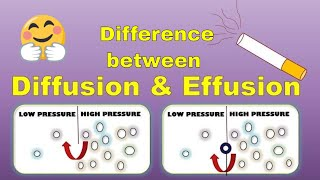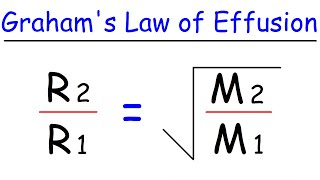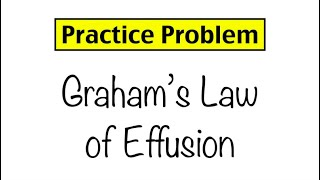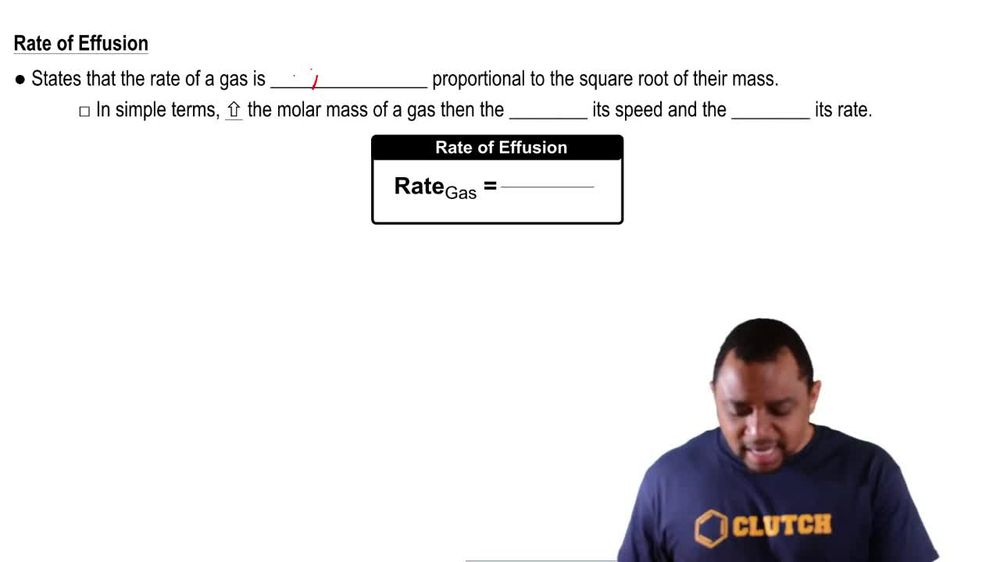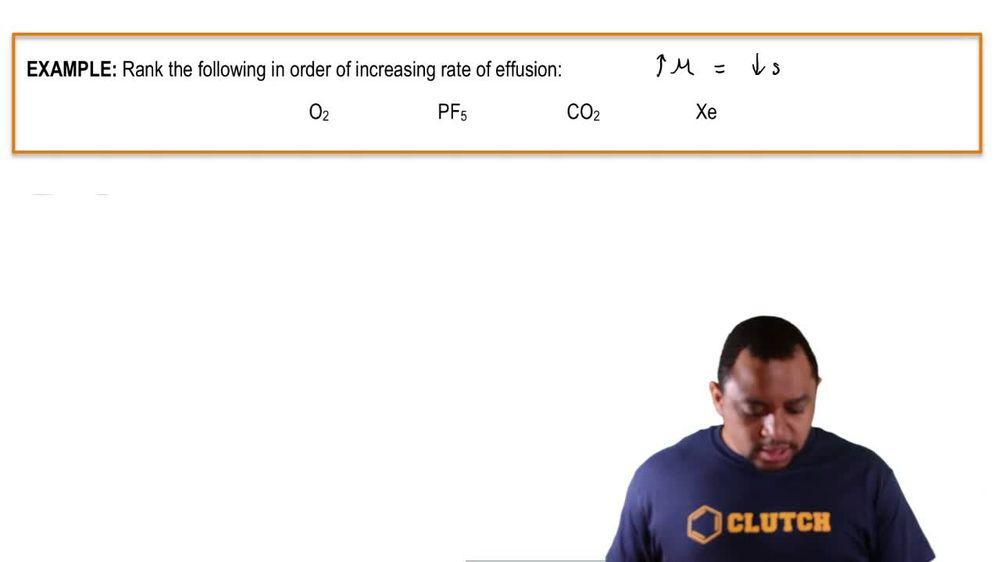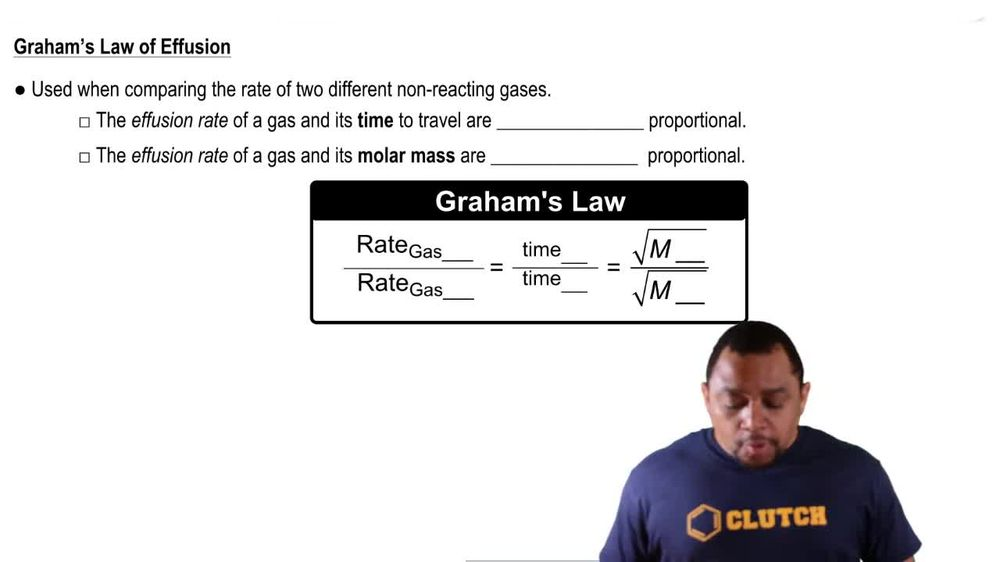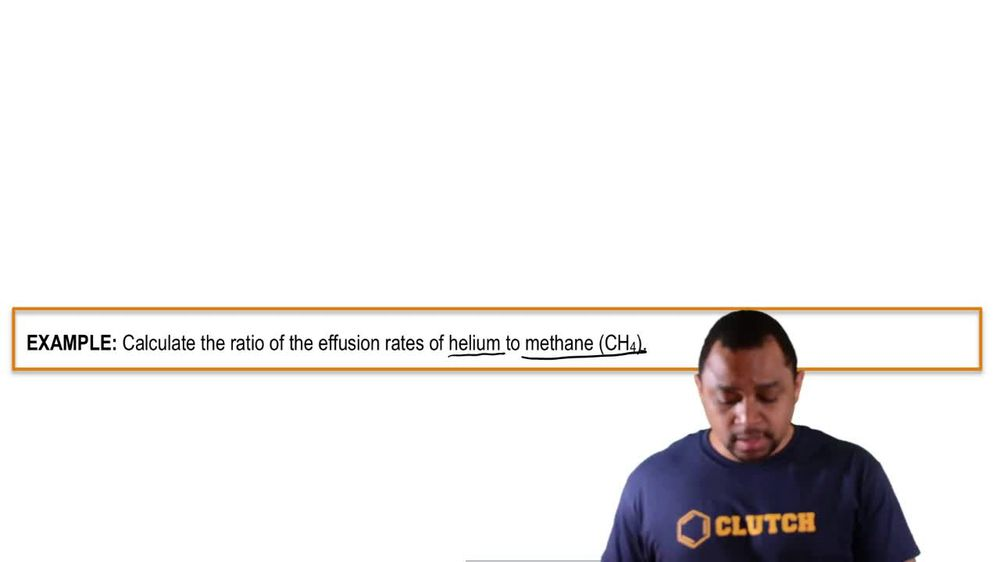Start typing, then use the up and down arrows to select an option from the list.
1. 7. Gases2. Effusion# Effusion

by Jules Bruno
133 views
2
0
now grands lava fusion is used when comparing the rate of two different non reacting gasses. And we're going to say here that the effusion rate of a gas and it's time to travel are directly proportional. So let's say that we're dealing with gas is A and B. So gas A and gas be since rate and time are directly proportional, that means they'll be on the same level. So the rate of gas A is equal to the time of gas. A. The rate of gas B is equal to the time of gas be. But then remember when we talked about the rate of a fusion? The fusion rate of a gas and it's Moeller mass are inversely proportional, so that would mean that the rate of gas A is on top. Therefore, the molar mass of gas. They would have to be on the bottom than the rate of gas B is here on the bottom and therefore it smaller mass has to be here on top. So remember, when we're directly proportional, we're on the same level with one another, and when we're inversely proportional, one will be numerator and the other will be the denominator. Understanding these relationships are key to answering questions dealing with Graham's law of a fusion